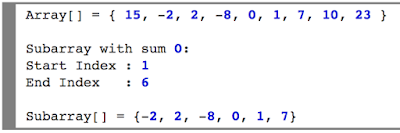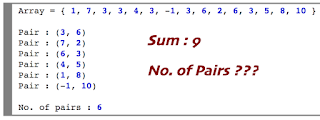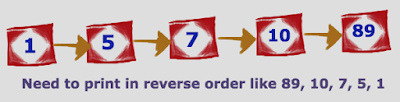Showing posts with label amazon interview questions. Show all posts
Showing posts with label amazon interview questions. Show all posts## Longest palindrome Sub-sequence from the given String using Dynamic Programming

Write a program to find the longest sub-sequence palindrome from the given string by using dynamic programming. For example

Input String : ABCDQRDC

Longest sub-sequence palindrome: 5

•  So let's see how we will solve and find the longest subsequence palindrome using dynamic programming.

• Construct the solution matrix of size NxN where N is the length of given string.
• We will calculate the size of each character sequence palindrome size and will the memorised in the solution matrix which will referred for the next incremental sequence.
• For example in above example all strings of 1 character will be palindrome size of 1. A, B, C, D, Q, R, D, C
• On next cycle it will add another character and makes 2 character string like
• AB, BC, CD, DQ, QR, RD, DC and goes on like
• ABC, BCD, CDQ, DQR, QRD, RDC etc.,
• Each sequences palindrome size will be stored in the solution array and finally returns the maximum size as output.
Now lets see simple Java code

``` public class LongestPalindromeSequence {

public static void main(String[] args) {

String str = "ABCDQRDC";

LongestPalindromeSequence obj = new LongestPalindromeSequence();

System.out.println("\nLength of Longest palindrome subsequence : " + obj.lps(str));

}

public int lps(String seq) {
int n = seq.length();
int j, k, i;
int L[][] = new int[n][n];

// Strings of 1 character will be palindrome size of 1
// starting with upper matrix and all single character falls diagonally and size will be 1
for (j = 0; j < n; j++)
L[j][j] = 1;

for (i = 2; i <= n; i++) {
for (j = 0; j < n - i + 1; j++) {

k = j + i - 1;

if (seq.charAt(j) == seq.charAt(k)) {
L[j][k] = L[j + 1][k - 1] + 2;

} else {
L[j][k] = Math.max(L[j][k - 1], L[j + 1][k]);
}
}
}

/*System.out.println("Final solution Matrix :::: ");
for (j = 0; j < n; j++) {
System.out.print(seq.charAt(j) +" - ");
for (k = 0; k < n; k++) {
System.out.print(L[j][k] + "\t");
}
System.out.println();
}*/
return L[n - 1];
}
}
```

OUTPUT:

```Length of Longest palindrome subsequence : 5
```## How to find missing number in a sequential array ?

Given a list of sequential integers and need to finding a missing number from the list of numbers. For example if we have an array like

Example:1
array = {1,2,3,5,6}
Finding missing number 4

Example:2
array = {14,15,16,18,19,20}
then the missing number is 17

Using XOR operation and finding the missing number.

• Iterate all the elements and do XOR with each other.
• Iterate all the numbers between given smallest and greatest number. In our above example between 1 to 6 and 14 to 20.
• Next do XOR with both results which gives the missing number

Lets see simple Java code implementation

```public class FindingMissingNumber {

public static void main(String[] args) {

int[] arr = new int[]{11,12,13,15,16,17,18,19};
int sr = 11;
int er = 19;

FindingMissingNumber obj = new FindingMissingNumber();

System.out.println("Missing number using XOR : "+obj.findMissingNumberUsingXOR(arr, sr, er));
}

public int findMissingNumberUsingXOR(int[] arr, int sr, int er){

int xor = 0;
int actualXor = 0;

for(int i=0;i<arr.length;i++){
xor = xor ^ arr[i];;
}

for(int i=sr;i<=er;i++){
actualXor = actualXor ^ i;
}

return (xor ^ actualXor);
}
}
```

OUTPUT:

```Missing number using XOR : 14
```## Split the array into two equal Sum subarrays

 Given an array of integers greater than zero, find it possible to split it in two subarrays such that the sum of the two subarrays is the same or with minimal difference. Print the two subarrays.

Examples:

Input: {5,7,9,2,69,23,9,1,8,5,7,3,6,16}

Output
1st Array sum  : 85
2nd Array sum : 85

ARRAY-1 : 69, 8, 7, 1,
ARRAY-2 : 23, 2, 16, 3, 9, 5, 9, 5, 6, 7,

Input: {1,2,3,4,5,6,7,8,9,10}

Output:
1st Array sum  : 27
2nd Array sum : 28

ARRAY-1 : 10, 8, 2, 6, 1,
ARRAY-2 : 9, 3, 7, 4, 5,

Lets see simple solution how to split the given array with equal sum or minimal difference.

Steps:
1. Sort the given array.
2. Iterate the array from both end and place the values in 2 different arraylist and also maintain the sum of both list.
3. On each iteration check for both sum difference and if they are not equal then swap difference value from 1st array to 2nd array or wise-verse to make it equal or minimal difference between both the array.
4. Finally print both the array sum and list of elements in both array.

Simple java code for the above solution:

```import java.util.ArrayList;
import java.util.List;

public class ArrayPartition {

public static void main(String[] args) {

int[] array = new int[] {5,7,9,2,69,23,9,1,8,5,7,3,6,16};
//int[] array = new int[] {1,2,3,4,5,6,7,8,9,10,1,3,3,6};
//int[] array = new int[] {5,5,2,2,1,1,2};
//int[] array = new int[] {1,2,3,4,5,6,7,8,9,10};
//int[] array = new int[] {100,50,55,2,2,1};
//int[] array = new int[] {1 , 2 , 3 , 6, 12};
//int[] array = new int[] {1,2,3,4};
//int[] array = new int[] {1000, 5000, 3000, 1000};
//int[] array = new int[] {1,2,3,4,5,6,7};
//int[] array = new int[] {1,1,1,1,1,1,2,4,2,2,1,3};
//int[] array = new int[] {10,100,50,110};
//int[] array = new int[] {4, 1, 2, 3};
//int[] array = new int[] {500000, 1,332234,3565, 332234};

// Sort the given array
sortArray(array);

// Call partition method to split the array
partition(array);

}

public static void partition(int[] array) {

List<Integer> arr1 = new ArrayList<Integer>();
List<Integer> arr2 = new ArrayList<Integer>();

int sum1 = 0, sum2 = 0;

// Place last value (largest) value in array1
sum1 = array[array.length-1];

// Iterate remaining values in array
for (int i=0,j=array.length-2;i<=j;) {

if(sum2 <= sum1) {
sum2 += array[i];
i++;
}else {
sum1 += array[i];
i++;
}

if(i <= j) {
if(sum2 <= sum1) {
sum2 += array[j];
j--;
}else {
sum1 += array[j];
j--;
}
}
}```
```   // Reorder array1 and array2 with equal sum or minimal sum
if(sum1 > sum2) {
int diff = (sum1 - sum2)/2;
if(arr1.contains(diff) && diff > 0) {
int sum[] = replaceArrayElement(arr1, diff, arr2, sum1, sum2);
sum1 = sum;
sum2 = sum;

}

while(sum1 > sum2 && diff > 0) {
diff--;
if(arr1.contains(diff) && diff > 0) {
int sum[] = replaceArrayElement(arr1, diff, arr2, sum1, sum2);
sum1 = sum;
sum2 = sum;
diff++;
}
}
}

// Reorder array1 and array2 with equal sum or minimal sum
if(sum2 > sum1) {
int diff = (sum2 - sum1)/2;
if(arr2.contains(diff) && diff > 0) {
int sum[] = replaceArrayElement(arr2, diff, arr1, sum2, sum1);
sum2 = sum;
sum1 = sum;
}

while(sum2 > sum1 && diff > 0) {
diff--;
if(arr2.contains(diff) && diff > 0) {
int sum[] = replaceArrayElement(arr2, diff, arr1, sum2, sum1);
sum2 = sum;
sum1 = sum;
diff++;
}
}
}

System.out.println("1st Array sum  : "+sum1);
System.out.println("2nd Array sum : "+sum2);

System.out.print("\nARRAY-1 : ");
for (Integer integer : arr1) {
System.out.print(integer +", ");
}

System.out.print("\nARRAY-2 : ");

for (Integer integer : arr2) {
System.out.print(integer +", ");
}
}

private static int[] replaceArrayElement(List<Integer> arr1, int diff, List<Integer> arr2, int sum1, int sum2) {

int in = arr1.indexOf(diff);
arr1.remove(in);
sum1 = sum1 - diff;
sum2 = sum2 + diff;

return new int[] {sum1, sum2};
}

private static void sortArray(int[] array) {

for(int i=0;i<=array.length-1;i++) {
for(int j=i+1;j<=array.length-1;j++) {
if(array[i] > array[j]) {
int tmp = array[i];
array[i] = array[j];
array[j] = tmp;
}
}
}
}
}
```

OUTPUT:

```1st Array sum  : 85
2nd Array sum : 85

ARRAY-1 : 69, 8, 7, 1,
ARRAY-2 : 23, 2, 16, 3, 9, 5, 9, 5, 6, 7,
```## How to do simple matrix multiplication

Simple matrix multiplication with sample java code.

```public class MatrixMultiplication {

public static void main(String[] args) {

int a[][] = new int[][] { {2,3}, {1,2}, {5,6} };
int b[][] = new int[][] { {4,5,6}, {1,2,3} };

if(a.length != b.length) {
System.out.println("Multiplication not possible");
return;
}

int c[][] = new int[a.length][b.length];

for(int i=0;i<a.length;i++)
for(int j=0;j<b.length;j++)
for(int k=0;k<a.length;k++)
c[i][j] += a[i][k] * b[k][j];

for(int i=0;i<a.length;i++) {
for(int j=0;j<b.length;j++)
System.out.print(c[i][j] + " ");
System.out.println();
}
}
}
```

OUTPUT:

```11   16    21
6    9     12
26   37   48
```## How to find the largest subarray with sum 0

Given an array of integers, find the largest subarray with sum equals to 0. If theres no subarray with sum 0 then print as "No subarray with sum 0".
As solution we are going to iterate the give array from 0th index to nth index and sum each values and finding the subarray.
Solution:
• Iterating from starting to end of the array
• Iterating sub array from 'start' to 'end' pointer and break if sum zero got or sub array smaller than previous sub array with zero
Lets see simple java code

```public class SubArrayZero {

public static void main(String[] args) {

int array[] = new int[] { 15, -2, 2, -8, 0, 1, 7, 10, 23 };

SubArrayZero obj = new SubArrayZero();

obj.getSubArraySumZero(array);
}

public void getSubArraySumZero(int[] array) {

int start = 0, end = 0, sum = 0, fStart = 1, fEnd = -1;

// Iterating from starting to end of the array
for (int i = 0; i < array.length; i++) {

end = i;
sum += array[i];

if (sum == 0) {
if ((fEnd - fStart) < (end - start)) {
fStart = start;
fEnd = end;
}
} else {
int tSum = sum;

/* Iterating sub array from 'start' to 'end' pointer and break
*  if sum zero got or sub array smaller than previous sub array with zero
*/
for (int j = start; j < end; j++) {
tSum -= array[j];
if (tSum == 0) {
if ((fEnd - fStart) < (end - (j + 1))) {
fStart = j + 1;
fEnd = end;
}
break;
} else if ((fEnd - fStart) > (end - (j + 1)))
break;
}
}
}
if(fEnd != -1) {
System.out.println("Start Index : " + fStart);
System.out.println("End Index   : " + fEnd);
}else {
System.out.println("No subarray with sum 0");
}
}
}
```

OUTPUT:

```Start Index : 1
End Index   : 6
```## How to find integer pairs with given sum

Given an array of integers, and a number ‘sum’, find the number of pairs of integer in the array whose sum is equal to ‘sum’.

Array can be a combination of +ve, -ve and duplicate elements. Where we need to get the list of all unique pairs from the given array which makes sum is equal to given number. Easy solution with O(N) time complexity will be
• Iterate each element from array and store it in Set.
• Compare the elements difference from sum as whether present in the Set or not. If present then print the pair with element and sum difference.
• Remove the difference element from Set to avoid generating duplicate pair.
Lets see simple Java solution

```import java.util.HashSet;

public class PairSum {

public static void main(String[] args) {

int[] val = new int[] { 1, 7, 3, 3, 3, 4, 3, -1, 3, 6, 6, 6, 6, 2, 6, 3, 3, 5, 8, 10 };
PairSum obj = new PairSum();
obj.getPairs(val, 9);
}

public void getPairs(int[] val, int sum) {

HashSet<Integer> set = new HashSet<Integer>();
int count = 0;
for (int i : val) {
if (set.contains(sum - i)) {
System.out.println("Pair : (" + (sum - i) + ", " + i + ")");
set.remove(sum - i); // Removing existing value from set to avoid duplicate pair to print
count++;
}
}

System.out.println("\nNo. of pairs : "+count);
}
}
```

OUTPUT:

```Pair : (3, 6)
Pair : (7, 2)
Pair : (6, 3)
Pair : (4, 5)
Pair : (1, 8)
Pair : (-1, 10)

No. of pairs : 6
```## How to print singly linked list in reverse order

If we talk about Singly Linked List then it will be a 1 way traversal from head node to tail node. But if we need to print the linked list values from tail node to head node (in reverse order) with O(N) time complexity. ???

• Its simple just we need to apply recursive algorithm where we need to start from head node and reach tail which is last node.
• Next print each node values in recursive call. By this way we can achieve printing linked list in recursive order without traversing more than once which is by time complexity O(N) only.
Lets see simple code to create Singly Linked List and printing same Linked List in reverse order with O(N) complexity.

```class NODE {

int data;
NODE next;

public NODE(int data) {
this.data = data;
this.next = null;
}
}

public static void main(String[] args) {

int val[] = new int[] { 1, 5, 7, 10, 89 };

}

/*
* Recursive method to print LL in reverse order
*/
public void printLLInReverse(NODE tmp) {
if (tmp != null) {
printLLInReverse(tmp.next);
System.out.println(tmp.data);
}
}

/*
* Create linked list based on given array
*/
NODE start = null;
for (int i : val) {
NODE tmp = new NODE(i);
if (start == null) {
start = tmp;
} else {
NODE mover = start;
while (mover.next != null) {
mover = mover.next;
}
mover.next = tmp;
}
}
return start;
}
}
```

OUTPUT:

```89
10
7
5
1
```## Finding Minimum Distinct Ids

Given an array of items, an i-th index element denotes the item id’s and given a number m, the task is to remove m elements such that there should be minimum distinct id’s left.Print the number of distinct id’s.
Input:
The first line of the input contains a single integer T, denoting the number of test cases. Then Ttest case follows, the three lines of the input, the first line contains N, denoting number of elements in an array,second line contains N elements/ids, and third line contains the number M.
Output:
For each test case, print the minimum number of distinct ids.
Constraints:
1<=T<=100
1<=N<=100
1<=arr[i]<=10^6
1<=M<=100
Example:
Input:

2
6
2 2 1 3 3 3
3
8
2 4 1 5 3 5 1 3
2
Output:
1
3

```import java.util.ArrayList;
import java.util.Arrays;
import java.util.Collections;
import java.util.Comparator;
import java.util.HashMap;
import java.util.List;
import java.util.Map;
import java.util.Scanner;
import java.util.Set;
import java.util.Map.Entry;

public class MinimumDistinct {

private static final Scanner scanner = new Scanner(System.in);
private static ArrayList<Integer> output = new ArrayList<Integer>();

public static void main(String[] args) {

int t = scanner.nextInt();
scanner.skip("(\r\n|[\n\r\u2028\u2029\u0085])?");

for (int i = 0; i < t; i++) {
int n = scanner.nextInt();
scanner.skip("(\r\n|[\n\r\u2028\u2029\u0085])?");

String array = scanner.nextLine();
scanner.skip("(\r\n|[\n\r\u2028\u2029\u0085])?");

int arr[] = Arrays.stream(array.split(" ")).mapToInt(Integer::parseInt).toArray();
int m = scanner.nextInt();
scanner.skip("(\r\n|[\n\r\u2028\u2029\u0085])?");

getMinimumDistinct(n, arr, m);
}

for (int ids : output) {
System.out.println(ids);
}
}

/*
* Convert array to map and get the distinct count
*/
private static void getMinimumDistinct(int n, int arr[], int m) {
HashMap<Integer, Integer> map = new HashMap<Integer, Integer>();
for (int i : arr) {
if(map.containsKey(i)) {
map.put(i, (map.get(i)+1));
}else {
map.put(i, 1);
}
}
sortMapAndGetDistinct(map , m);

}

/*
* Sort the map based on value and remove the least numbers of ids for m times
*/
private static void sortMapAndGetDistinct(HashMap<Integer, Integer> map, int m) {
Set<Entry<Integer, Integer>> set = map.entrySet();
List<Entry<Integer, Integer>> list = new ArrayList<Entry<Integer, Integer>>(set);
Collections.sort( list, new Comparator<Map.Entry<Integer, Integer>>() {
public int compare( Map.Entry<Integer, Integer> o1, Map.Entry<Integer, Integer> o2 ) {
return (o1.getValue()).compareTo( o2.getValue() );
}
} );
for(Map.Entry<Integer, Integer> entry:list){
if(m > 0 && entry.getValue() <= m) {
map.remove(entry.getKey());
m = m-entry.getValue();
}
}
}
}
```

INPUT:
```2
6
2 2 1 3 3 3
3
8
2 4 1 5 3 5 1 3
2
```

OUTPUT:
```1
3
```